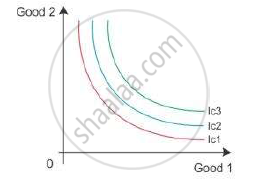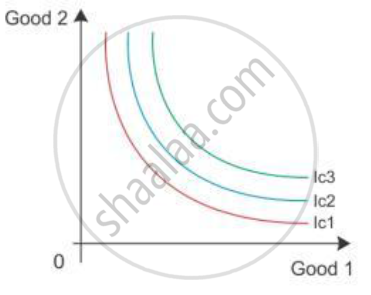# Define an Indifference Curve. Explain Why an Indifference Curve is Downward Sloping from Left to Right. - Economics

Define an indifference curve.

What is Indifference Curve?

#### Solution 1

An indifference map refers to a set of indifference curves which represent consumer preferences over all the bundles of two goods. An indifference curve shows all the combinations which create the same level of satisfaction. We can present an indifference curve with the high or low level of satisfaction, i.e. to the right and above another show a higher level of satisfaction to the consumer. Here, IC3 shows the higher level of satisfaction than IC2. Thus, the indifference curve also relates to a higher level of income of the consumer. However, all these curves have the same level of satisfaction.#### Solution 2

An indifference curve shows all the combinations which create the same level of satisfaction. We can present an indifference curve with the high or low level of satisfaction, i.e. to the right and above another show a higher level of satisfaction to the consumer. Here, IC3 shows the higher level of satisfaction than IC2.Concept: Indifference Curve
Is there an error in this question or solution?
2011-2012 (March) Delhi Set 1
Share